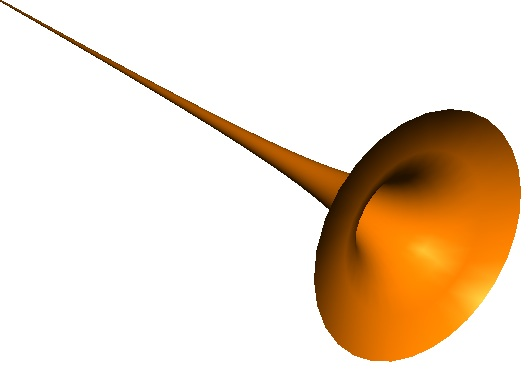# Paint the horn

Calculus Level 4A hypothetical horn is constructed, which is described by the parameters $(r,\theta)$ as

$H=\left\{(x,y,z)|x=\frac{\cos(\theta)}{r},y=\frac{\sin(\theta)}{r},z=-r,r\in (1,\infty),\theta \in (0,2\pi)\right\}$

If it is required to paint the interior of the horn. If 100 square units of the interior surface can be covered using one cubic unit of paint, of the available options what is the minimum number of cubic units of paint that would ensure that the entire interior of the horn is covered in paint.

×